# dsp.HighpassFilter

FIR or IIR highpass filter

## Description

The `dsp.HighpassFilter` System object™ independently filters each channel of the input over time using the given design specifications. You can set the `FilterType` property of `dsp.HighpassFilter` to `'FIR'` or `'IIR'` to implement the object as an FIR or IIR highpass filter.

When the `FilterType` property is set to `'FIR'`, using this object is an alternative to using the `firceqrip` and `firgr` functions with `dsp.FIRFilter`. The `dsp.HighpassFilter` object condenses the two-step process into one. You can use `measure` to verify that the design meets the prescribed specifications.

To filter each channel of your input:

1. Create the `dsp.HighpassFilter` object and set its properties.

2. Call the object with arguments, as if it were a function.

This object supports C/C++ code generation and SIMD code generation under certain conditions. This object also supports code generation for ARM® Cortex®-M and ARM Cortex-A processors. For more information, see Code Generation.

## Creation

### Syntax

``HPF = dsp.HighpassFilter``
``HPF = dsp.HighpassFilter(Name=Value)``

### Description

````HPF = dsp.HighpassFilter` returns a minimum order FIR highpass filter, `HPF`, with the default filter settings. Calling the object with the default property settings filters the input data with a stopband frequency of `8` kHz, a passband frequency of `12` kHz, a stopband attenuation of `80` dB, and a passband ripple of `0.1` dB.```

example

````HPF = dsp.HighpassFilter(Name=Value)` returns a highpass filter with additional properties specified by one or more `Name-Value` pair arguments. `Name` is the property name and `Value` is the corresponding value. For example, `StopbandFrequency=8000` sets the stopband frequency specification of the filter to 8000 Hz.```

## Properties

expand all

Unless otherwise indicated, properties are nontunable, which means you cannot change their values after calling the object. Objects lock when you call them, and the `release` function unlocks them.

If a property is tunable, you can change its value at any time.

Filter type, specified as one of these options:

• `'FIR'` — The object designs an FIR highpass filter.

• `'IIR'` — The object designs an IIR highpass (biquad) filter.

Flag to design minimum order filter, specified as:

• `true` –– The object designs the minimum order filter that meets the filter design specifications.

• `false` –– The object designs the filter with the order that you specify in the `FilterOrder` property.

Order of the FIR or IIR filter, specified as a positive integer.

#### Dependencies

To enable this property, set `DesignForMinimumOrder` to `false`.

Data Types: `single` | `double` | `int8` | `int16` | `int32` | `int64` | `uint8` | `uint16` | `uint32` | `uint64`

Filter stopband edge frequency, specified as a real positive scalar in Hz or in normalized frequency units (since R2023a).

If you set the `NormalizedFrequency` property to:

• `false` –– The value of the stopband edge frequency is in Hz. The value must be less than the passband edge frequency and half the `SampleRate` property value.

• `true` –– The value of the stopband edge frequency is in normalized frequency units. The value must be a positive scalar less than the passband edge frequency and less than `1.0`.

(since R2023a)

#### Dependencies

To enable this property, set the `DesignForMinimumOrder` property to `true`.

Data Types: `single` | `double` | `int8` | `int16` | `int32` | `int64` | `uint8` | `uint16` | `uint32` | `uint64`

Filter passband edge frequency, specified as a real positive scalar in Hz or in normalized frequency units (since R2023a).

If you set the `NormalizedFrequency` property to:

• `false` –– The value of the passband edge frequency is in Hz. The value must be less than half the `SampleRate` property value and greater than the `StopbandFrequency` property value.

• `true` –– The value of the passband edge frequency is in normalized frequency units. The value must be a positive scalar less than `1.0` and greater than the `StopbandFrequency` property value.

(since R2023a)

Data Types: `single` | `double` | `int8` | `int16` | `int32` | `int64` | `uint8` | `uint16` | `uint32` | `uint64`

Minimum attenuation in the stopband, specified as a real positive scalar in dB.

Data Types: `single` | `double` | `int8` | `int16` | `int32` | `int64` | `uint8` | `uint16` | `uint32` | `uint64`

Maximum ripple of filter response in the passband, specified as a real positive scalar in dB.

Data Types: `single` | `double` | `int8` | `int16` | `int32` | `int64` | `uint8` | `uint16` | `uint32` | `uint64`

Since R2023a

Flag to set frequencies in normalized units, specified as one of these values:

• `true` –– The passband edge and stopband edge frequencies must be in the normalized frequency units and less than `1.0`.

• `false` –– The passband edge and stopband edge frequencies are in Hz. You can specify the input sample rate through the `SampleRate` property.

Data Types: `logical`

Input sample rate in Hz, specified as a positive real scalar.

#### Dependency

To enable this property, set `NormalizedFrequency` to `false`. (since R2023a)

Data Types: `single` | `double`

Fixed-Point Properties

Rounding method for output fixed-point operations, specified as a character vector. For more information on the rounding modes, see Precision and Range.

Word and fraction lengths of coefficients, specified as a `numerictype` object. The default, `numerictype(1,16)` corresponds to a signed numeric type object with 16-bit coefficients and a fraction length determined based on the coefficient values, to give the best possible precision.

This property is not tunable.

Word length of the output is same as the word length of the input. Fraction length of the output is computed such that the entire dynamic range of the output can be represented without overflow. For details on how the fraction length of the output is computed, see Fixed-Point Precision Rules for Avoiding Overflow in FIR Filters.

## Usage

### Syntax

``y = HPF(x)``

### Description

example

````y = HPF(x)` highpass filters the input signal, `x`. `y` is a highpass-filtered version of `x`.```

### Input Arguments

expand all

Noisy data input, specified as a vector or a matrix. If the input signal is a matrix, each column of the matrix is treated as an independent channel. The number of rows in the input signal denote the channel length. This object accepts variable-size inputs. After the object is locked, you can change the size of each input channel, but you cannot change the number of channels.

Data Types: `single` | `double` | `int8` | `int16` | `int32` | `int64` | `uint8` | `uint16` | `uint32` | `uint64` | `fi`
Complex Number Support: Yes

### Output Arguments

expand all

Filtered output, returned as a vector or a matrix. The output has the same size, data type, and complexity characteristics as the input.

Data Types: `single` | `double` | `int8` | `int16` | `int32` | `int64` | `uint8` | `uint16` | `uint32` | `uint64` | `fi`
Complex Number Support: Yes

## Object Functions

To use an object function, specify the System object as the first input argument. For example, to release system resources of a System object named `obj`, use this syntax:

`release(obj)`

expand all

 `freqz` Frequency response of discrete-time filter System object `fvtool` Visualize frequency response of DSP filters `impz` Impulse response of discrete-time filter System object `info` Information about filter System object `coeffs` Returns the filter System object coefficients in a structure `cost` Estimate cost of implementing filter System object `grpdelay` Group delay response of discrete-time filter System object `outputDelay` Determine output delay of single-rate or multirate filter `generatehdl` Generate HDL code for quantized DSP filter (requires Filter Design HDL Coder) `measure` Measure frequency response characteristics of filter System object
 `step` Run System object algorithm `release` Release resources and allow changes to System object property values and input characteristics `reset` Reset internal states of System object

## Examples

collapse all

Create a minimum order FIR highpass filter for data sampled at 44.1 kHz. Specify a passband frequency of 12 kHz, a stopband frequency of 8 kHz, a passband ripple of 0.1 dB, and a stopband attenuation of 80 dB.

```Fs = 44.1e3; filtertype = 'FIR'; Fpass = 12e3; Fstop = 8e3; Rp = 0.1; Astop = 80; FIRHPF = dsp.HighpassFilter(SampleRate=Fs,... FilterType=filtertype,... PassbandFrequency=Fpass,... StopbandFrequency=Fstop,... PassbandRipple=Rp,... StopbandAttenuation=Astop);```

Design a minimum order IIR highpass filter with the same properties as the FIR highpass filter. Use `clone` to create a system object with the same properties as the FIR Highpass filter. Change the `FilterType` property of the cloned filter to `IIR`.

```IIRHPF = clone(FIRHPF); IIRHPF.FilterType = 'IIR';```

Plot the impulse response of the FIR highpass filter. The zeroth order coefficient is delayed by 19 samples, which is equal to the group delay of the filter. The FIR highpass filter is a causal FIR filter

`fvtool(FIRHPF,Analysis='impulse')`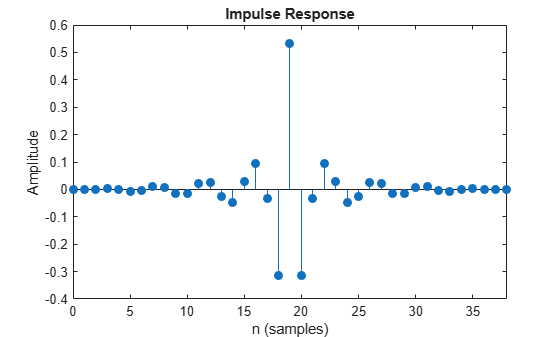Plot the impulse response of the IIR highpass filter.

`fvtool(IIRHPF,Analysis='impulse')`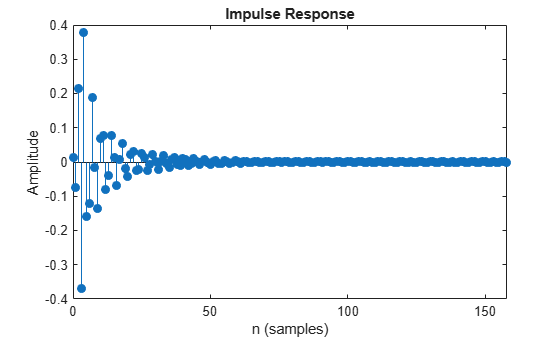Plot the magnitude and phase response of the FIR highpass filter.

`fvtool(FIRHPF,Analysis='freq')`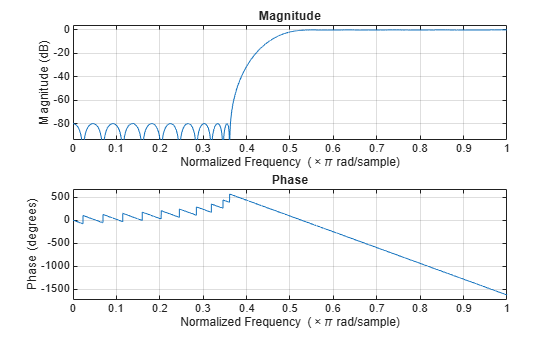Plot the magnitude and phase response of the IIR highpass filter.

`fvtool(IIRHPF,Analysis='freq')`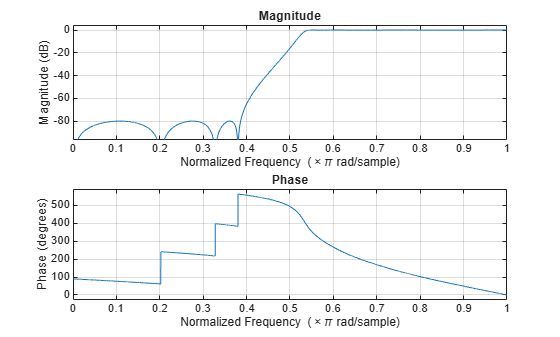Calculate the cost of implementing the FIR highpass filter.

`cost(FIRHPF)`
```ans = struct with fields: NumCoefficients: 39 NumStates: 38 MultiplicationsPerInputSample: 39 AdditionsPerInputSample: 38 ```

Calculate the cost of implementing the IIR highpass filter. The IIR filter is more efficient to implement than its FIR counterpart.

`cost(IIRHPF)`
```ans = struct with fields: NumCoefficients: 18 NumStates: 14 MultiplicationsPerInputSample: 18 AdditionsPerInputSample: 14 ```

Calculate the group delay of the FIR highpass filter.

`grpdelay(FIRHPF)`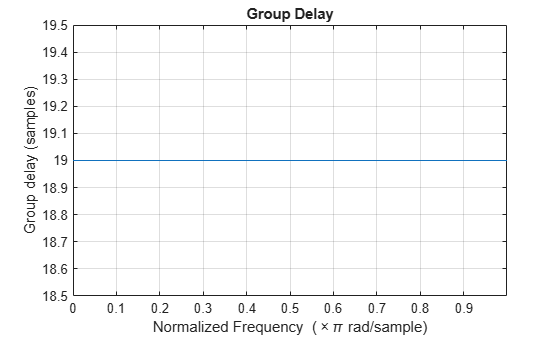Calculate the group delay of the IIR highpass filter. The FIR filter has a constant group delay (linear phase) while its IIR counterpart does not.

`grpdelay(IIRHPF)`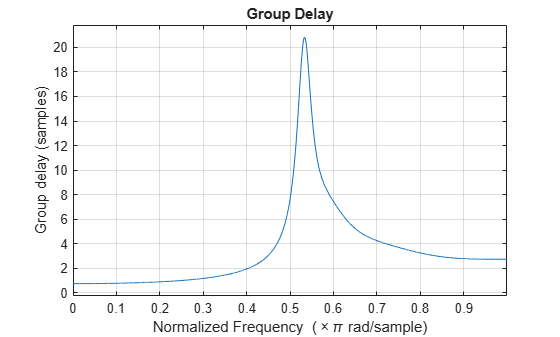Create a highpass filter using the `dsp.HighpassFilter` object. Setting the `NormalizedFrequency` property to `true` designs the filter with frequency specifications in normalized frequency units.

`LPF = dsp.HighpassFilter(NormalizedFrequency=true)`
```LPF = dsp.HighpassFilter with properties: FilterType: 'FIR' DesignForMinimumOrder: true StopbandFrequency: 0.3628 PassbandFrequency: 0.5442 StopbandAttenuation: 80 PassbandRipple: 0.1000 NormalizedFrequency: true Use get to show all properties ```

Create a `spectrumAnalyzer` object to visualize the input and output signal spectra. With a sample rate of 44.1e3 Hz, the stopband frequency and the passband frequency of the filter translate to 8000 Hz and 12000 Hz, respectively.

```SA = spectrumAnalyzer(SampleRate=44.1e3,... PlotAsTwoSidedSpectrum=false,ShowLegend=true,... YLimits=[-150 30],... Title='Input Signal and Output Signal of Lowpass Filter'); SA.ChannelNames = {'Input','Output'};```

Run the highpass filter algorithm to filter the white Gaussian noisy input signal. View the input and output signals using the spectrum analyzer.

```for k = 1:100 Input = randn(1024,1); Output = LPF(Input); SA([Input,Output]); end```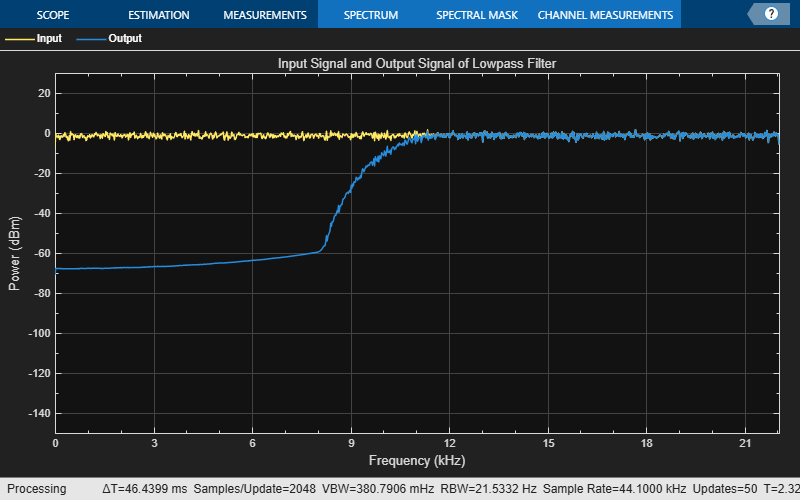Set up the IIR highpass filter. The sampling rate of the white Gaussian noise is 44,100 Hz. The passband frequency of the filter is 12 kHz, the stopband frequency is 8 kHz, the passband ripple is 0.1 dB, and the stopband attenuation is 80 dB.

```Fs = 44.1e3; filtertype = 'IIR'; Fpass = 12e3; Fstop = 8e3; Rp = 0.1; Astop = 80; hpf = dsp.HighpassFilter(SampleRate=Fs,... FilterType=filtertype,... PassbandFrequency=Fpass,... StopbandFrequency=Fstop,... PassbandRipple=Rp,... StopbandAttenuation=Astop);```

View the magnitude response of the highpass filter.

`fvtool(hpf)`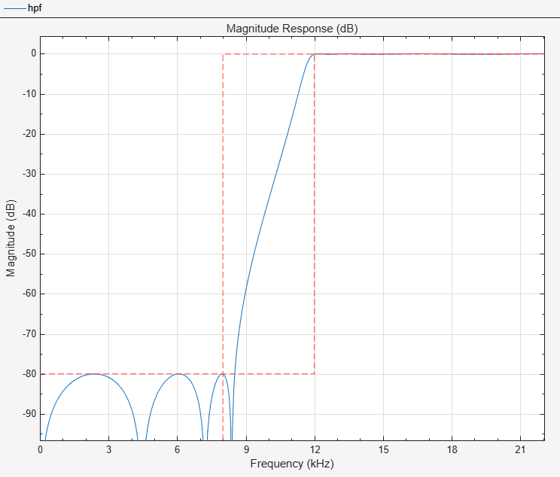Create a spectrum analyzer object.

```sa = spectrumAnalyzer(SampleRate=44.1e3,... PlotAsTwoSidedSpectrum=false,ShowLegend=true,... YLimits=[-150 30],... Title='Input Signal and Output Signal of IIR Highpass Filter'); sa.ChannelNames = {'Input','Output'};```

Filter the white Gaussian noisy input signal. View the input and output signals using the spectrum analyzer.

```for k = 1:100 Input = randn(1024,1); Output = hpf(Input); sa([Input,Output]); end```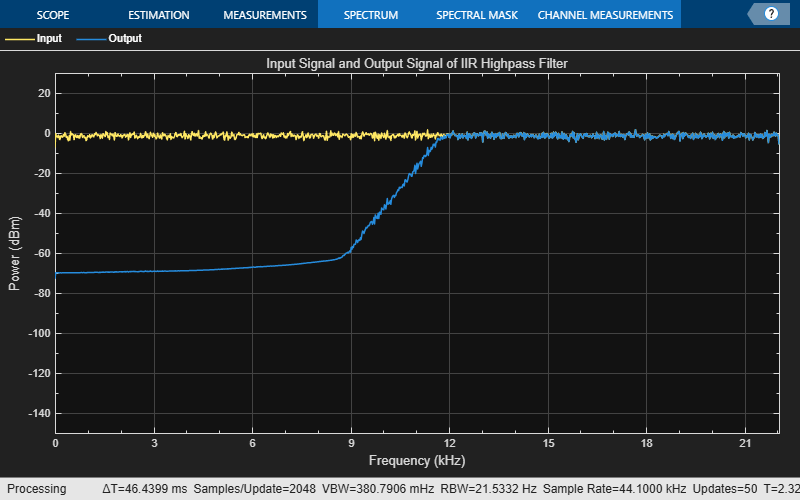Measure the frequency response characteristics of a highpass filter. Create a `dsp.HighpassFilter` System object with default properties. Measure the frequency response characteristics of the filter.

`HPF = dsp.HighpassFilter`
```HPF = dsp.HighpassFilter with properties: FilterType: 'FIR' DesignForMinimumOrder: true StopbandFrequency: 8000 PassbandFrequency: 12000 StopbandAttenuation: 80 PassbandRipple: 0.1000 NormalizedFrequency: false SampleRate: 44100 Use get to show all properties ```
`HPFMeas = measure(HPF)`
```HPFMeas = Sample Rate : 44.1 kHz Stopband Edge : 8 kHz 6-dB Point : 10.418 kHz 3-dB Point : 10.8594 kHz Passband Edge : 12 kHz Stopband Atten. : 81.8558 dB Passband Ripple : 0.08066 dB Transition Width : 4 kHz ```

## Algorithms

expand all

 Shpak, D.J., and A. Antoniou. "A generalized Remez method for the design of FIR digital filters." IEEE® Transactions on Circuits and Systems. Vol. 37, Issue 2, Feb. 1990, pp. 161–174.

 Selesnick, I.W., and C. S. Burrus. "Exchange algorithms that complement the Parks-McClellan algorithm for linear-phase FIR filter design." IEEE Transactions on Circuits and Systems. Vol. 44, Issue 2, Feb. 1997, pp. 137–143.